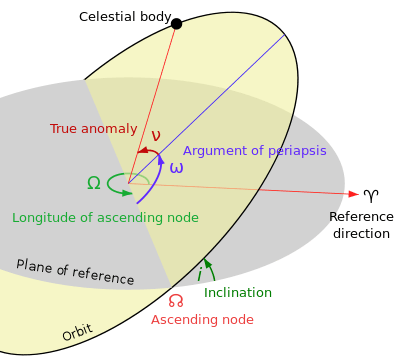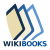# Orbital elements

Orbital elements are the parameters required to uniquely identify a specific orbit. In celestial mechanics these elements are generally considered in classical two-body systems, where a Kepler orbit is used (derived from Newton's laws of motion and Newton's law of universal gravitation). There are many different ways to mathematically describe the same orbit, but certain schemes, each consisting of a set of six parameters, are commonly used in astronomy and orbital mechanics.

A real orbit (and its elements) changes over time due to gravitational perturbations by other objects and the effects of relativity. A Keplerian orbit is merely an idealized, mathematical approximation at a particular time.

## Keplerian elementsIn this diagram, the orbital plane (yellow) intersects a reference plane (gray). For Earth-orbiting satellites, the reference plane is usually the Earth's equatorial plane, and for satellites in solar orbits it is the ecliptic plane. The intersection is called the line of nodes, as it connects the center of mass with the ascending and descending nodes. This plane, together with the vernal point (), establishes a reference frame.

The traditional orbital elements are the six Keplerian elements, after Johannes Kepler and his laws of planetary motion.

When viewed from an inertial frame, two orbiting bodies trace out distinct trajectories. Each of these trajectories has its focus at the common center of mass. When viewed from a non-inertial frame centred on one of the bodies, only the trajectory of the opposite body is apparent; Keplerian elements describe these non-inertial trajectories. An orbit has two sets of Keplerian elements depending on which body is used as the point of reference. The reference body is called the primary, the other body is called the secondary. The primary does not necessarily possess more mass than the secondary, and even when the bodies are of equal mass, the orbital elements depend on the choice of the primary.

The main two elements that define the shape and size of the ellipse:

• Eccentricity (e)—shape of the ellipse, describing how much it is elongated compared to a circle (not marked in diagram).
• Semimajor axis (a)—the sum of the periapsis and apoapsis distances divided by two. For circular orbits, the semimajor axis is the distance between the centers of the bodies, not the distance of the bodies from the center of mass.

Two elements define the orientation of the orbital plane in which the ellipse is embedded:

• Inclination (i)—vertical tilt of the ellipse with respect to the reference plane, measured at the ascending node (where the orbit passes upward through the reference plane, the green angle i in the diagram). Tilt angle is measured perpendicular to line of intersection between orbital plane and reference plane. Any three points on an ellipse will define the ellipse orbital plane. The plane and the ellipse are both two-dimensional objects defined in three-dimensional space.
• Longitude of the ascending node ( or Ω)—horizontally orients the ascending node of the ellipse (where the orbit passes upward through the reference plane) with respect to the reference frame's vernal point (the green angle Ω in the diagram).

And finally:

• Argument of periapsis (ω) defines the orientation of the ellipse in the orbital plane, as an angle measured from the ascending node to the periapsis (the closest point the satellite object comes to the primary object around which it orbits, the blue angle ω in the diagram).
• True anomaly (ν, θ, or f) at epoch (M0) defines the position of the orbiting body along the ellipse at a specific time (the "epoch").

The mean anomaly is a mathematically convenient "angle" which varies linearly with time, but which does not correspond to a real geometric angle. It can be converted into the true anomaly ν, which does represent the real geometric angle in the plane of the ellipse, between periapsis (closest approach to the central body) and the position of the orbiting object at any given time. Thus, the true anomaly is shown as the red angle ν in the diagram, and the mean anomaly is not shown.

The angles of inclination, longitude of the ascending node, and argument of periapsis can also be described as the Euler angles defining the orientation of the orbit relative to the reference coordinate system.

Note that non-elliptic trajectories also exist, but are not closed, and are thus not orbits. If the eccentricity is greater than one, the trajectory is a hyperbola. If the eccentricity is equal to one and the angular momentum is zero, the trajectory is radial. If the eccentricity is one and there is angular momentum, the trajectory is a parabola.

### Required parameters

Given an inertial frame of reference and an arbitrary epoch (a specified point in time), exactly six parameters are necessary to unambiguously define an arbitrary and unperturbed orbit.

This is because the problem contains six degrees of freedom. These correspond to the three spatial dimensions which define position (x, y, z in a Cartesian coordinate system), plus the velocity in each of these dimensions. These can be described as orbital state vectors, but this is often an inconvenient way to represent an orbit, which is why Keplerian elements are commonly used instead.

Sometimes the epoch is considered a "seventh" orbital parameter, rather than part of the reference frame.

If the epoch is defined to be at the moment when one of the elements is zero, the number of unspecified elements is reduced to five. (The sixth parameter is still necessary to define the orbit; it is merely numerically set to zero by convention or "moved" into the definition of the epoch with respect to real-world clock time.)

### Alternative parametrizations

Keplerian elements can be obtained from orbital state vectors (three vectors for the position and three for the velocity) by manual transformations or with computer software.

Other orbital parameters can be computed from the Keplerian elements such as the period, apoapsis, and periapsis. (When orbiting the Earth, the last two terms are known as the apogee and perigee.) It is common to specify the period instead of the semi-major axis in Keplerian element sets, as each can be computed from the other provided the standard gravitational parameter, GM, is given for the central body.

Instead of the mean anomaly at epoch, the mean anomaly M, mean longitude, true anomaly ν0, or (rarely) the eccentric anomaly might be used.

Using, for example, the "mean anomaly" instead of "mean anomaly at epoch" means that time t must be specified as a seventh orbital element. Sometimes it is assumed that mean anomaly is zero at the epoch (by choosing the appropriate definition of the epoch), leaving only the five other orbital elements to be specified.

Different sets of elements are used for various astronomical bodies. The eccentricity, e, and either the semi-major axis, a, or the distance of periapsis, q, are used to specify the shape and size of an orbit. The angle of the ascending node, Ω, the inclination, i, and the argument of periapsis, ω, or the longitude of periapsis, ϖ, specify the orientation of the orbit in its plane. Either the longitude at epoch, L0, the mean anomaly at epoch, M0, or the time of perihelion passage, T0, are used to specify a known point in the orbit. The choices made depend whether the vernal equinox or the node are used as the primary reference. The semi-major axis is known if the mean motion and the gravitational mass are known.

It is also quite common to see either the mean anomaly (M) or the mean longitude (L) expressed directly, without either M0 or L0 as intermediary steps, as a polynomial function with respect to time. This method of expression will consolidate the mean motion (n) into the polynomial as one of the coefficients. The appearance will be that L or M are expressed in a more complicated manner, but we will appear to need one fewer orbital element.

Mean motion can also be obscured behind citations of the orbital period P.

Sets of orbital elements
Object Elements used
Major planet e, a, i, Ω, ϖ, L0
Comet e, q, i, Ω, ω, T0
Asteroid e, a, i, Ω, ω, M0
Two-line elements e, i, Ω, ω, n, M0

#### Euler angle transformations

The angles Ω, i, ω are the Euler angles (α, β, γ with the notations of that article) characterizing the orientation of the coordinate system

, ŷ, from the inertial coordinate frame Î, Ĵ,

where:

• Î, Ĵ is in the equatorial plane of the central body. Î is in the direction of the vernal equinox. Ĵ is perpendicular to Î and with Î defines the reference plane. is perpendicular to the reference plane.
• , ŷ are in the orbital plane and with in the direction to the pericenter (periapsis). is perpendicular to the plane of the orbit. ŷ is mutually perpendicular to and .

Then, the transformation from the Î, Ĵ, coordinate frame to the , ŷ, frame with the Euler angles Ω, i, ω is:whereThe transformation from , ŷ, to Euler angles Ω, i, ω is:where arg(x,y) signifies the polar argument that can be computed with the standard function atan2(y,x) available in many programming languages.

## Orbit prediction

Under ideal conditions of a perfectly spherical central body and zero perturbations, all orbital elements except the mean anomaly are constants. The mean anomaly changes linearly with time, scaled by the mean motion,Hence if at any instant t0 the orbital parameters are [e0, a0, i0, Ω0, ω0, M0], then the elements at time t0 + δt is given by [e0, a0, i0, Ω0, ω0, M0 + n δt]

## Perturbations and elemental variance

Unperturbed, two-body, Newtonian orbits are always conic sections, so the Keplerian elements define an ellipse, parabola, or hyperbola. Real orbits have perturbations, so a given set of Keplerian elements accurately describes an orbit only at the epoch. Evolution of the orbital elements takes place due to the gravitational pull of bodies other than the primary, the nonsphericity of the primary, atmospheric drag, relativistic effects, radiation pressure, electromagnetic forces, and so on.

Keplerian elements can often be used to produce useful predictions at times near the epoch. Alternatively, real trajectories can be modeled as a sequence of Keplerian orbits that osculate ("kiss" or touch) the real trajectory. They can also be described by the so-called planetary equations, differential equations which come in different forms developed by Lagrange, Gauss, Delaunay, Poincaré, or Hill.

## Two-line elements

Main article: Two-line elements

Keplerian elements parameters can be encoded as text in a number of formats. The most common of them is the NASA/NORAD "two-line elements" (TLE) format, originally designed for use with 80-column punched cards, but still in use because it is the most common format, and can be handled easily by all modern data storages as well.

Depending on the application and object orbit, the data derived from TLEs older than 30 days can become unreliable. Orbital positions can be calculated from TLEs through the SGP/SGP4/SDP4/SGP8/SDP8 algorithms.

Example of a two-line element:

 1 27651U 03004A   07083.49636287  .00000119  00000-0  30706-4 0  2692
2 27651 039.9951 132.2059 0025931 073.4582 286.9047 14.81909376225249


## References

1. For example, with VEC2TLE
2. Green, Robin M. (1985). Spherical Astronomy. Cambridge University Press. ISBN 978-0-521-23988-2.
3. Danby, J. M. A. (1962). Fundamentals of Celestial Mechanics. Willmann-Bell. ISBN 978-0-943396-20-0.
4. Kelso, T.S. "CelesTrak: "FAQs: Two-Line Element Set Format"". celestrak.com. Retrieved 15 June 2016.
5. Explanatory Supplement to the Astronomical Almanac. 1992. K. P. Seidelmann, Ed., University Science Books, Mill Valley, California.
6. SORCE - orbit data at Heavens-Above.com
• Gurfil, Pini (2005). "Euler parameters as nonsingular orbital elements in Near-Equatorial Orbits". J. Guid. Contrl. Dynamics. 28 (5). doi:10.2514/1.14760.Wikibooks has a book on the topic of: Astrodynamics/Classical Orbit Elements